## 切削速度(vc)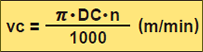※用1000去除，为将mm换算成m

vc (m/min);切削速度
DC (mm):铣刀直径
π (3.14):圆周率

n (min-1):主轴转速

DC(mm)
n(min-1)
vc(m/min)

### 例题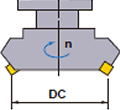### 答

π=3.14、DC=125、n=350代入公式
vc=(π×DC×n)÷1000=(3.14×125×350)÷1000
=137.4(m/min)

## 每刃进给量 (fz)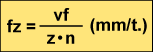fz (mm/t.):每刃进给量
vf (mm/min):每分钟工作台进给速度
n (min-1):

z:刃数

vf(mm/min)
z
n(min-1)
fz(mm/t.)

### 例题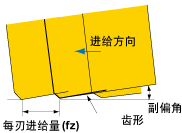### 答

fz=Vf÷(z×n)=500÷(10×500)=0.1mm/t.

## 工作台进给速度 (vf)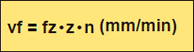vf (mm/min):每刃进给量
vf (mm/min):每分钟工作台进给速度
n (min-1):主轴转速 (每转进给量 f=zxfz)
z:刃数

### 例题### 答

vf=fz×z×n=0.1×10×500=500mm/min

## 加工时间(Tc)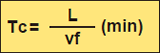Tc (min):加工时间
vf (mm/min):每分钟工作台进给速度
L (mm):

L(mm)
vf(mm/min)
Tc(min)

### 例题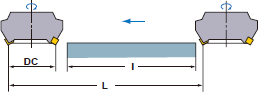### 答

vf=0.25×16×200=800mm/min

L=300+200=500mm

Tc=500÷800=0.625(min)
0.625×60=约37.5秒